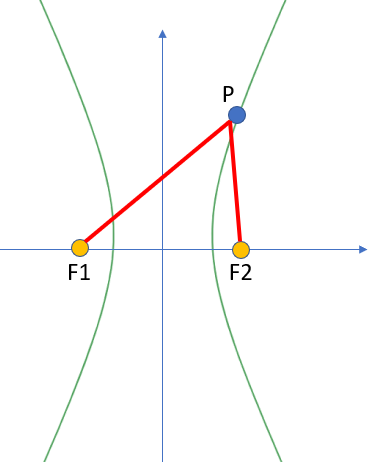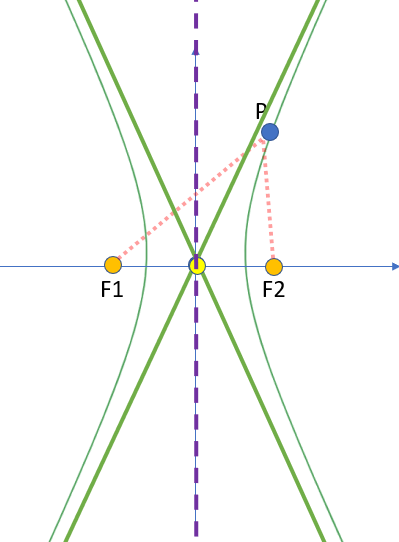# Hyperbola: Definition, Examples, Equations

A hyperbola is an open curve with two opposite, mirror-image parabolas.The difference of the distance from point P to foci F1 and F2 on a hyperbola is constant.
More formally, it is a collection of points in the plane where the absolute value of the difference of the distances from a point on the hyperbola to each foci is a fixed constant. Another way to put this: point P on the curve shown above is always closer to F2 than F1 by a fixed amount. If point P were on the left-hand curve (instead of the right), then point P would always be closer to F1 than F2 by the same fixed amount. As a formula:

|P(F1) – P(F2)| = constant.

Parts of a hyperbola:

• The branches are the two continuous curves.
• The two lines connected the focal points F1 and F2 are the focal radii at point P.
• The midpoint is the center of the curves, located halfway between point F1 and F2. If the parabola is centered at the origin, then the origin is the midpoint.
• The asympototes show where the curve is headed. Although these are not technically part of the shape, they can be helpful with sketching the shape.
• The axis of symmetry splits the two branches exactly down the middle.The midpoint (yellow dot) is halfway between F1 and F2. The asymptotes are shown as green lines and the purple line is the axis of symmetry.

If the hyperbola is centered at the origin with its foci on the x-axis (as in the above image), the equation is:If the foci are on the y-axis, the equation is:The equation can also be formatted as a second degree equation with two variables :
Ax2 – Cy2 + Dx + Ey + F = 0 or
-Ax2 – Cy2 + Dx + Ey + F = 0.

## Hyperbola and Conic Sections

The hyperbola, along with the ellipse and parabola, make up the conic sections. You can get a hyperbola by slicing through a double cone. The difference between this shape and a run-of-the-mill parabola is that the slice is steeper. The slice doesn’t have to be parallel to the cone’s axis, but it does have to create symmetrical curves .## References

 Bonds, D. Math 155, Lecture Notes-Bonds. Section 10.1 Conics and Calculus.
 Hyperbola. Retrieved July 30, 2021 from: http://jwilson.coe.uga.edu/EMT668/EMAT6680.F99/Kim/emat6690/instructional%20unit/hyperbola/Hyperbola/Hyperbola.htm

CITE THIS AS:
Stephanie Glen. "Hyperbola: Definition, Examples, Equations" From StatisticsHowTo.com: Elementary Statistics for the rest of us! https://www.statisticshowto.com/hyperbola-definition-examples-equations/